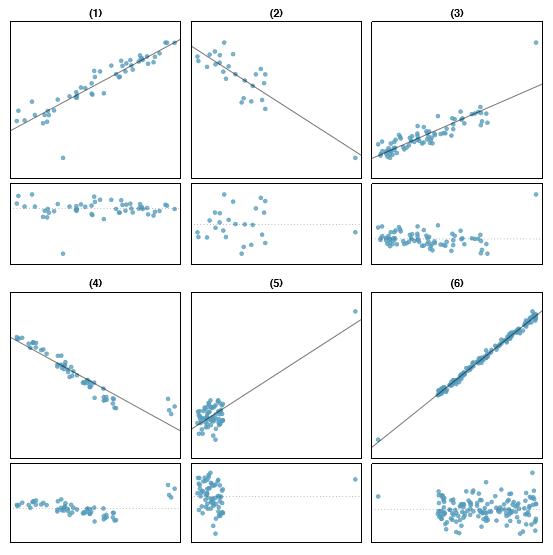# 7.3: Types of Outliers in Linear Regression

In this section, we identify criteria for determining which outliers are important and influential. Outliers in regression are observations that fall far from the "cloud" of points. These points are especially important because they can have a strong influence on the least squares line.

Example $$\PageIndex{1}$$

There are six plots shown in Figure $$\PageIndex{1}$$ along with the least squares line and residual plots. For each scatter plot and residual plot pair, identify any obvious outliers and note how they influence the least squares line. Recall that an outlier is any point that doesn't appear to belong with the vast majority of the other points.

1. There is one outlier far from the other points, though it only appears to slightly influence the line.
2. There is one outlier on the right, though it is quite close to the least squares line, which suggests it wasn't very influential.
3. There is one point far away from the cloud, and this outlier appears to pull the least squares line up on the right; examine how the line around the primary cloud doesn't appear to t very well.
4. There is a primary cloud and then a small secondary cloud of four outliers. The secondary cloud appears to be influencing the line somewhat strongly, making the least square line t poorly almost everywhere. There might be an interesting explanation for the dual clouds, which is something that could be investigated.
5. There is no obvious trend in the main cloud of points and the outlier on the right appears to largely control the slope of the least squares line.
6. There is one outlier far from the cloud, however, it falls quite close to the least squares line and does not appear to be very influential.

Examine the residual plots in Figure $$\PageIndex{1}$$. You will probably nd that there is some trend in the main clouds of (3) and (4). In these cases, the outliers influenced the slope of the least squares lines. In (5), data with no clear trend were assigned a line with a large trend simply due to one outlier (!).Figure $$\PageIndex{1}$$: Six plots, each with a least squares line and residual plot. All data sets have at least one outlier.

Definition: Leverage

Points that fall horizontally away from the center of the cloud tend to pull harder on the line, so we call them points with high leverage.

Points that fall horizontally far from the line are points of high leverage; these points can strongly influence the slope of the least squares line. If one of these high leverage points does appear to actually invoke its influence on the slope of the line (as in cases (3), (4), and (5) of Example $$\PageIndex{1}$$) then we call it an influential point. Usually we can say a point is influential if, had we plotted the line without it, the influential point would have been unusually far from the least squares line.

It is tempting to remove outliers. Do not do this without a very good reason. Models that ignore exceptional (and interesting) cases often perform poorly. For instance, if a financial firm ignored the largest market swings - the "outliers" - they would soon go bankrupt by making poorly thought-out investments.

Caution: Don't ignore outliers when fitting a final model

If there are outliers in the data, they should not be removed or ignored without a good reason. Whatever final model is fit to the data would not be very helpful if it ignores the most exceptional cases.

Caution: Outliers for a categorical predictor with two levels

Be cautious about using a categorical predictor when one of the levels has very few observations. When this happens, those few observations become inuential points.

## Contributors

David M Diez (Google/YouTube), Christopher D Barr (Harvard School of Public Health), Mine Çetinkaya-Rundel (Duke University)